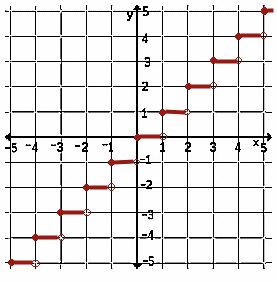Piece-Wise Defined Functions Break the Domain, Break the FunctionThe Absolute Value FunctionThe Greatest Integer Function (A Step Function)Break the Domain, Break the Functionrange, f(x) domain f(x) =-x/2 if x< 0 2sin(x) if 0 < x < 4 - 1.51 if x > 4

This is a typical piece-wise defined function.

The name piece-wise is used to indicated that the function is pieced together, or put together using other functions.

The colors help one see the parts. Examine the graph, its labels, and the definition of the function below it.

The green function piece is a line. Because its domain is x < 0, it is used for negative values of x and when x is 0.

The purple function piece is a sine curve. Because its domain is 0 < x<4, it is used for values of x between 0 and 4, including 4.

The blue function piece is a constant function, a horizontal line. Because its domain is x > 4, it is used for values of x greater than 4.

The Absolute Value FunctionThe absolute value function, | x |, need not be written as a piece-wise function. It is really the composition the square root of the square of a number,(x²).

Thought of as one piece, not many, the domain is all numbers.

If it is considered as pieces of other functions, the domain must also be stated in pieces.range, f(x) domain f(x) =-x if x< 0 x if x > 0

Defined as a piece-wise function, the absolute value is shown here in green and blue. Each piece is a line. Each piece has its own domain.

Some times the absolute value is written as a piece-wise function using 3 pieces.

 f(x) =-x if x< 0 0 if x = 0 x if x > 0

The absolute value is easy to represent. Sometimes it is more difficult to represent the function symbolically. See the greatest integer function, in the next box.

 The Greatest Integer Function (A Step Function)Think of steps. Think of paying sales tax, or income tax, or buying postage stamps.       Think of paying a nice round number for all services or numbers up to the next round number.       Think of the greatest integer less than or equal to the number in question. That's the greatest integer function.       Its symbol is [[x]]. Its graph looks like steps.       The function has discontinuities, breaks, going from one "step" to the next. In each interval, the lower endpoint is included (as shown by a colored in circle) and the upper endpoint (as shown by a hollow circle) is not included because it is included in the next "step."© 2008, Agnes Azzolino www.mathnstuff.com/math/spoken/here/2class/230/piecew.htm